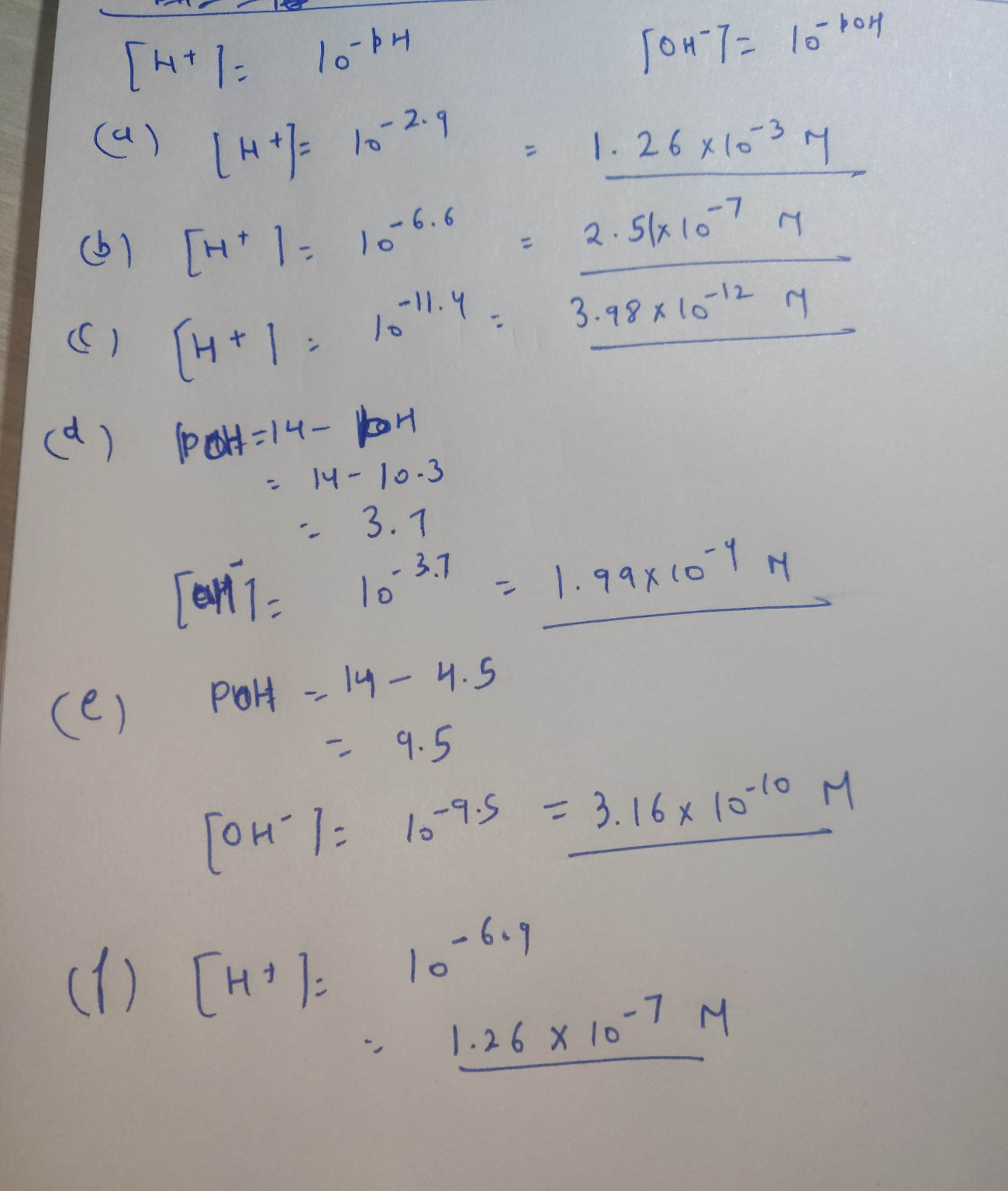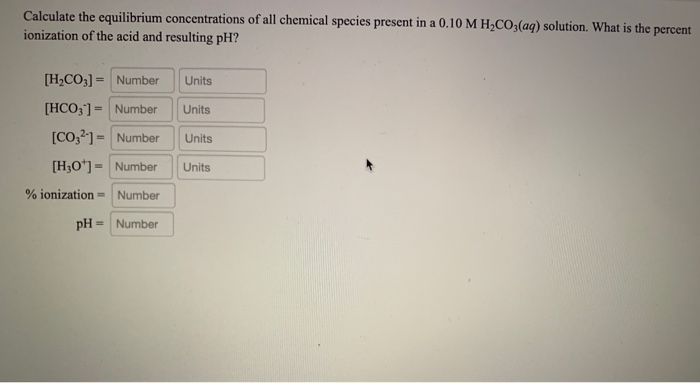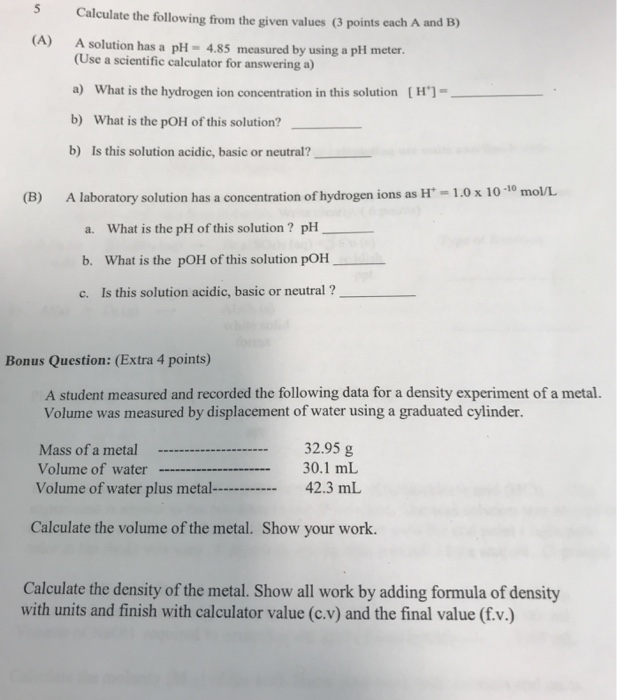Question

From the given PH values calculate the following: (including units)

a.) [H+] in vinegar with the pH is measured to be 2.9.

b.) [H+] in saliva if the pH is measured to be 6.6.

c.) [H+] in household ammonia, NH3, with a pH of 11.4

d.) [OH-] in milk of magnesia having a pH of 10.3

e.) [OH-] in beer with a pH of 4.5

f.) [H+] inside of liver cell if the pH is 6.9#### Earn Coins

Coins can be redeemed for fabulous gifts.

Similar Homework Help Questions
• ### Calculate the equilibrium concentrations of all chemical species present in a 0.10 M H CO.(aq) solution....Calculate the equilibrium concentrations of all chemical species present in a 0.10 M H CO.(aq) solution. What is the percent ionization of the acid and resulting pH? Units Units [H2CO3) = Number [HCO3) = Number [CO32) = Number [H30+) = Number Units Units % ionization = Number pH = Number Ka Acid Acetic Ammonium Formula CH3COOH NH4+ H3B03 1.8 x 10-5 5.6 x 10-10 5.4 x 10-10 Boric Carbonic H2CO3 Chlorous Formic Hydrocyanic Perchloric HCIO2 HCOOH HCN HC104 4.5 x...

• ### 5 Calculate the following from the given values (3 points each A and B) A) A...5 Calculate the following from the given values (3 points each A and B) A) A solution has a pH- 4.85 measured by using a phi meter (Use a scientific calculator for answering a) a) What is the hydrogen ion concentration in this solution [H'] b) What is the pOH of this solution? b) Is this solution acidic, basic or neutral? (B) A laboratory solution has a concentration of hydrogen ions as H-1.0x 10-10 mol/L a. What is the pH...